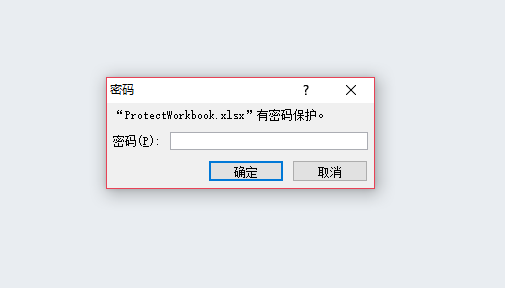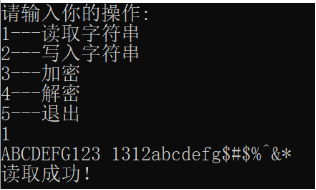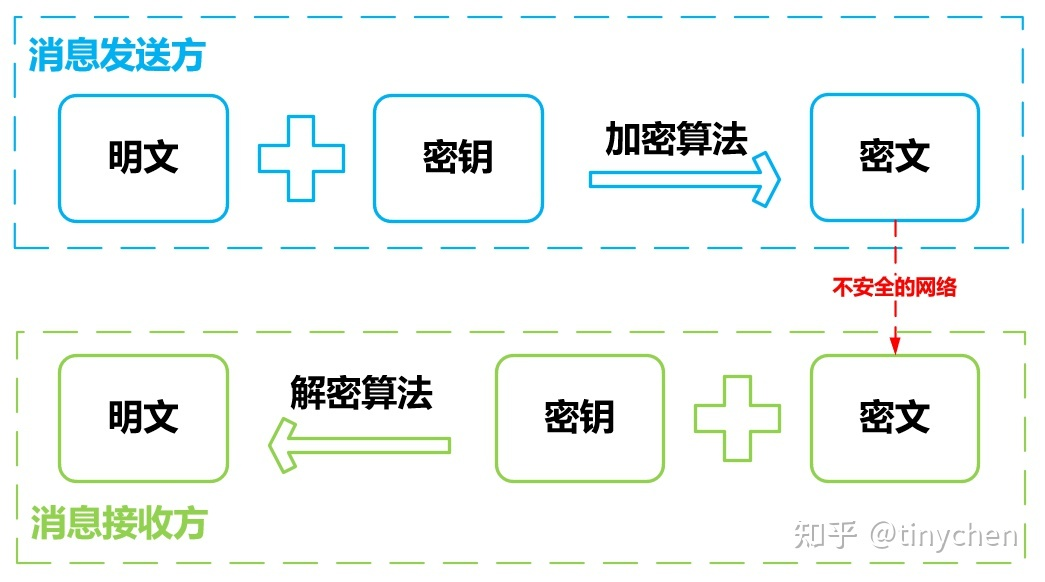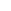# PHP加密解密原理-日常操作

04-30 531阅读

1、非常给力的加密函数，!经典代码（带详解）：

(\$,\$='',\$key='',\$=0){

//动态密匙长度，相同的明文会生成不同密文就是依靠动态密匙

\$=4;

//密匙

\$key=md5(\$key?\$key:\$['']);

//密匙a会参与加解密

\$keya=md5((\$key,0,16));

//密匙b会拿来做数据完整性验证

\$keyb=md5((\$key,16,16));

//密匙c用于变化生成的密文

\$keyc=\$?(\$==''?(\$,0,\$):

(md5(()),-\$)):'';

//参与运算的密匙

\$=\$keya.md5(\$keya.\$keyc);\$=(\$);

//明文，前10位用来保存时间戳，解密时验证数据有效性，10到26位用来保存\$keyb(密匙b)，

//解密时会借助这个密匙验证数据完整性

//假如是解码的话，会从第\$位开始，因为密文前\$位保存动态密匙，以确保解密正确

\$=\$==''?((\$,\$)):

('0d',\$?\$+time():0).(md5(\$.\$keyb),0,16).\$;

\$=(\$);

\$='';

\$box=range(0,255);

\$=array();

//产生密匙簿

for(\$i=0;\$i0)&&

(\$,10,16)==(md5((\$,26).\$keyb),0,16)){

(\$,26);

}else{

'';

}

}else{

//把动态密匙保存在密文里，这只是为什么同样的明文，生产不同密文后能解读的理由

//因为加密后的密文可能是一些特殊字符，复制过程或许会丢失php源码 未加密 文章，所以用编码\$keyc.('=','',(\$));

}

}

\$str='';

\$key='#39;;

echo(\$str,'',\$key,0);//加密

\$str='';echo(\$str,'',\$key,0);//解密

2、加解密函数()：

(\$,\$,\$key=''){

\$key=md5(\$key);

\$=(\$key);

\$=\$=='D'?(\$):(md5(\$.\$key),0,8).\$;

\$=(\$);

\$=\$box=array();

\$='';

for(\$i=0;\$i

• 1.注意：本站资源多为网络收集，如涉及版权问题请及时与站长联系，我们会在第一时间内删除资源。
• 2.您购买的只是资源，不提供解答疑问和安装服务。免费源码里的接口不保证一直可以用
• 3.本站用户发帖仅代表本站用户个人观点，并不代表本站赞同其观点和对其真实性负责。
• 4.本站资源大多存储在云盘，如发现链接失效，请及时与站长联系，我们会第一时间更新。
• 5.转载本网站任何内容，请按照转载方式正确书写本站原文地址
• 6.如果发现侵权可以联系站长删除站长QQ3326096692或者邮箱通知3326096692@qq.com
• #### 发表评论

快捷回复： 表情：
评论列表 （暂无评论，531人围观）

## 还没有评论，来说两句吧...

### 目录[+]请先 登录 再评论，若不是会员请先 注册